# FORM FOUR MATHEMATICS NOTES TOPIC 5: TRIGONOMETRY

Posted on

### FORM FOUR MATHEMATICS STUDY NOTES  TOPIC 5: TRIGONOMETRYTRIGONOMETRY

Trigonometric Ratios
The Sine, Cosine and Tangent of an Angle Measured in the Clockwise and Anticlockwise Directions
Determine the sine, cosine and tangent of an angle measured in the clockwise and anticlockwise directions
The basic three trigonometrical ratios are sine, cosine and tangent which are written in short as Sin, Cos, and tan respectively.
Consider the following right angled triangle.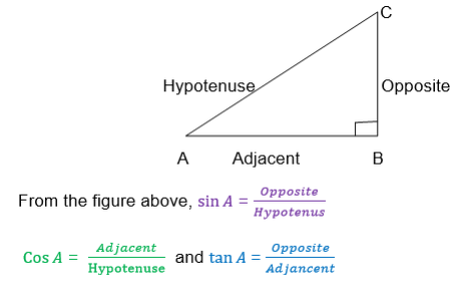Also we can define the above triangle ratios by using a unit Circle centered at the origin.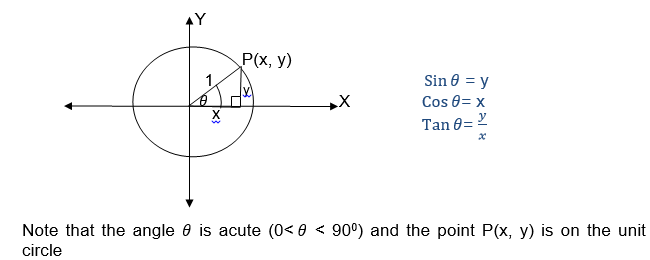If θis an obtuse angle (900<θ<1800) then the trigonometrical ratios are the same as the trigonometrical ratio of 1800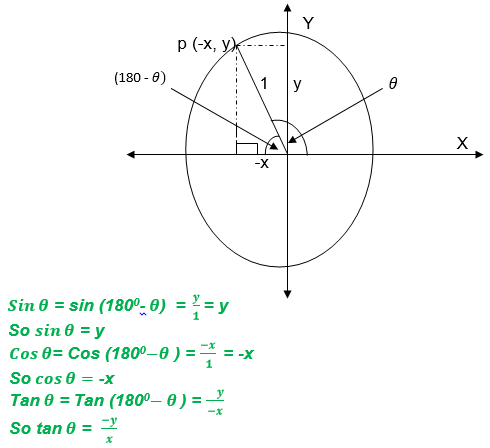If θis a reflex angle (1800< θ<2700) then the trigonometrical ratios are the same as that of θ- 1800If θis a reflex angle (2700< θ< 3600), then the trigonometrical ratios are the same as that of 360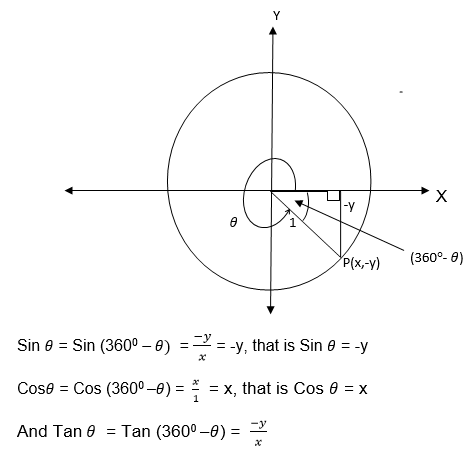We have seen that trigonometrical ratios are positive or negative depending on the size of the angle and the quadrant in which it is found.
The result can be summarized by using the following diagram.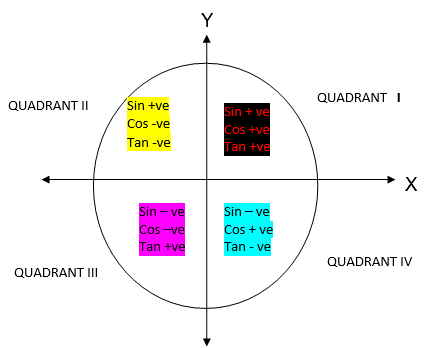Trigonometric Ratios to Solve Problems in Daily Life
Apply trigonometric ratios to solve problems in daily life
Example 1
Write the signs of the following ratios
1. Sin 1700
2. Cos 2400
3. Tan 3100
4. sin 300
Solution
a)Sin 1700
Since 1700 is in the second quadrant, then Sin 170= Sin (1800-1700) = Sin 100
∴Sin 1700 = Sin 100
b) Cos 2400 = -Cos (2400-1800)= -Cos 600
Therefore Cos 2400= -Cos 600
c) Tan 310= -Tan (3600-3100) = – Tan 500
Therefore Tan 3100= -Tan 500
d) Sin 3000= -sin (3600-3000) = -sin 600
Therefore sin 3000= – Sin 600
Relationship between Trigonometrical ratios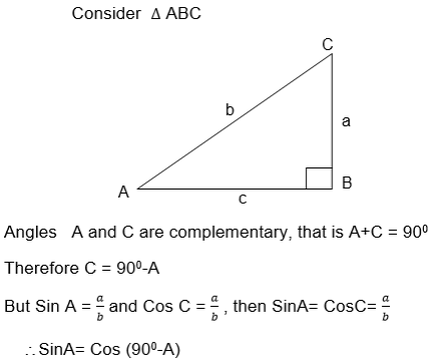The above relationship shows that the Sine of angle is equal to the cosine of its complement.
Also from the triangle ABC above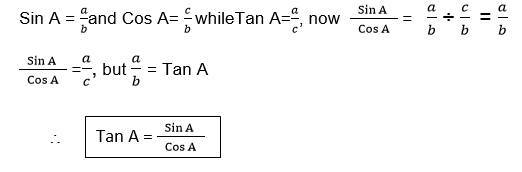Again using the ΔABC
b2 = a2+c2 (Pythagoras theorem)
And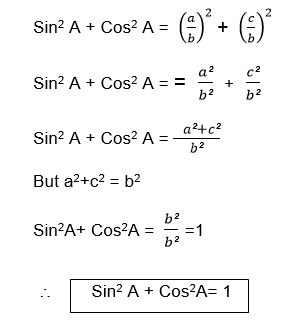Example 2
Given thatA is an acute angle and Cos A= 0.8, find
1. Sin A
2. tan A.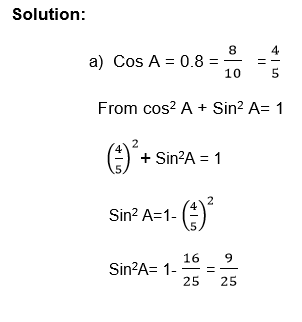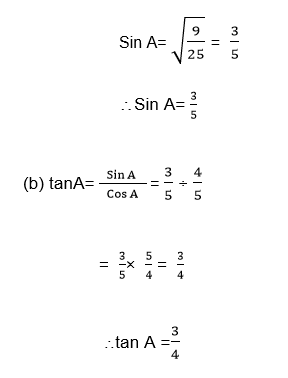Example 3
If A and B are complementary angles,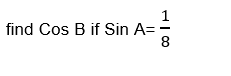Solution
If A and B are complementary angle
Then Sin A = Cos B and Sin B = Cos A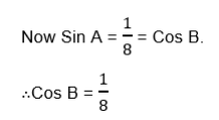Example 4
Given that θand βare acute angles such that θ+ β= 900 and Sinθ= 0.6, find tanβ
Solution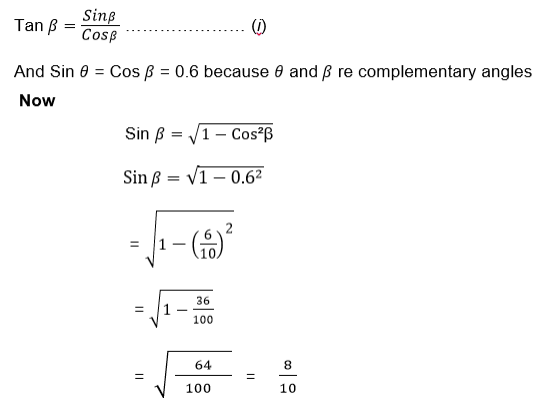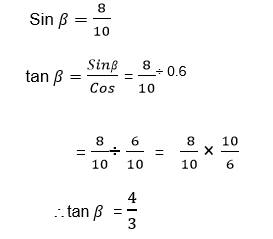Exercise 1
For practice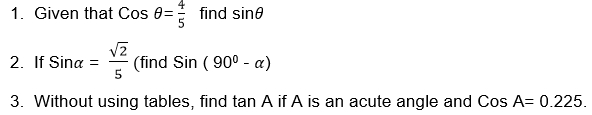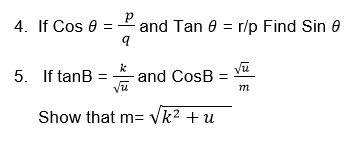The Compound of Angle Formulae or Sine, Cosine and Tangent in Solving Trigonometric Problems
Apply the compound of angle formulae or sine, cosine and tangent in solving trigonometric problems
The aim is to express Sin (α±β) and Cos (α±β) in terms of Sinα, Sinβ, Cosαand Cosβ
Consider the following diagram: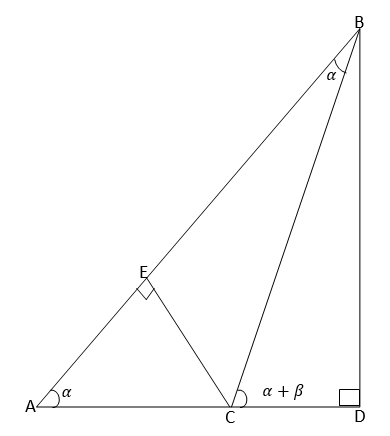From the figure above <BAD=αand <ABC=βthus<BCD=α+β
From ΔBCD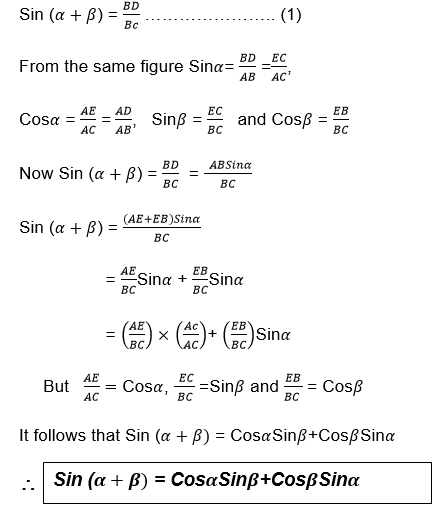For Cos(α±β) Consider the following unit circle with points P and Q on it such that OP,makes angleα with positive x-axis and OQ makes angle βwith positive x-axes.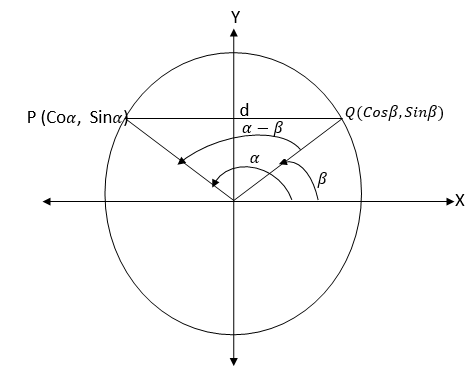From the figure above the distance d is given by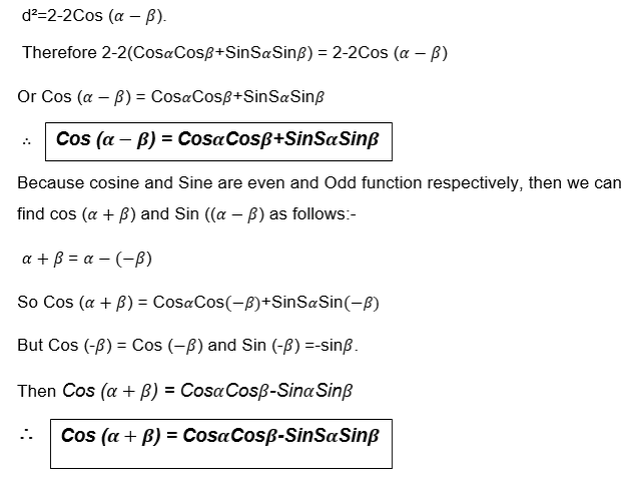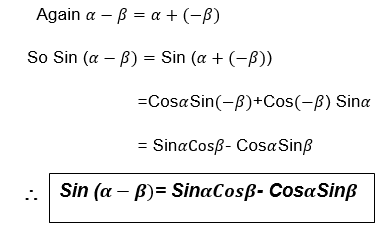In general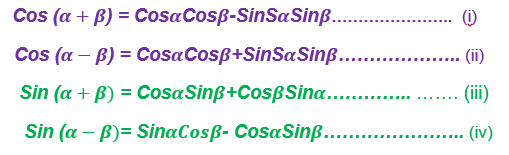Example 15
1. Withoutusing tables find the value of each of the following:
1. Sin 75°
2. Cos105
Solution: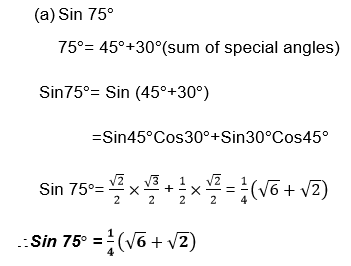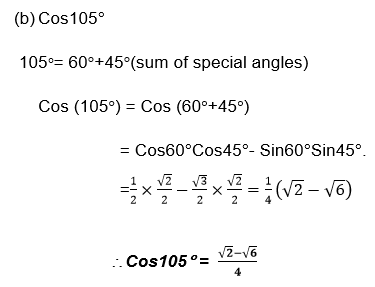Example 16
Find:
1. Sin150°
2. Cos 15°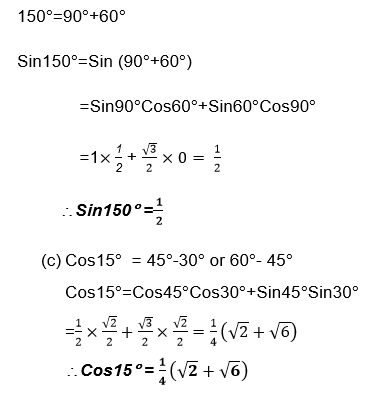Exercise 4
1. Withoutusing tables, find:
1. Sin15°
2. Cos 120°
2. FindSin 225° from (180°+45°)
3. <!–[endif]–>Verify that
1. Sin90° = 1 by using the fact that 90°=45°+45°
2. Cos90°=0 by using the fact that 90°=30°+60°
4. <!–[endif]–>Express each of the following in terms of sine, cosine and tangent of acute angles.
1. Sin107°
2. Cos300°
5. <!–[endif]–>By using the formula for Sin (A-B), show that Sin (90°-C)=Cos C